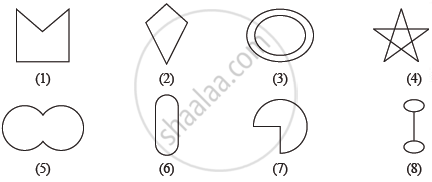Share

# Given Here Are Some Figures Classify Each of Them on the Basis of the Following Simple Curve, Simple Closed Curve, Polygon, Convex Polygon, Concave Polygon - Mathematics

Course

#### Question

Given here are some figuresClassify each of them on the basis of the following

(a) Simple curve

(b) Simple closed curve

(c) Polygon

(d) Convex polygon

(e) Concave polygon

#### Solution

Simple curve - 1, 2, 5, 6, 7

Simple closed curve - 1, 2, 5, 6, 7

Polygon - 1, 2

Convex polygon - 2

Concave polygon - 1

Is there an error in this question or solution?